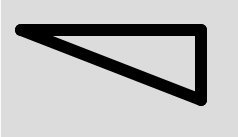# p5.js | strokeJoin() function

• Last Updated : 31 Oct, 2019

The strokeJoin() function in p5.js is used to set the joint style which connect the line segments. These joints are either mitered, beveled, or rounded and specified with the corresponding parameters MITER, BEVEL, and ROUND. MITER is the default joint.

Syntax:

`strokeJoin(join)`

Parameters: This function accepts single parameter join which stores the joint parameter constant either ‘MITER’, ‘BEVEL’ or ‘ROUND’.

Below programs illustrates the strokeJoin() function in p5.js:

Example 1: This example uses strokeJoin() function to join the strokes.

 `function` `setup() {``     ` `    ``// Create Canvas of given size``    ``createCanvas(380, 170);``}`` ` `function` `draw() {``     ` `    ``// Set the background color``    ``background(220);``     ` `    ``// Set the stroke weight``    ``strokeWeight(12);``     ` `    ``// Set strokeJoin function``    ``strokeJoin(ROUND);``     ` `    ``// Function to create line segment``    ``line(20, 30, 200, 30);``    ``line(200, 30, 200, 100);``    ``line(200, 100, 20, 30);``}`

Output:Example 2: This example uses strokeJoin() function to join the strokes.

 `function` `setup() {``     ` `    ``// Create Canvas of given size``    ``createCanvas(380, 170);``}`` ` `function` `draw() {``     ` `    ``// Set the background color``    ``background(220);``     ` `    ``// Set the stroke weight``    ``strokeWeight(10);``     ` `    ``// Set strokeJoin function``    ``strokeJoin(BEVEL);``     ` `    ``// Function to create line segment``    ``line(20, 30, 200, 30);``    ``line(200, 30, 200, 100);``    ``line(200, 100, 20, 100);``    ``line(20, 100, 20, 30);``}`

Output:My Personal Notes arrow_drop_up